## Cycle Sort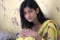Updated on 15-Jun-2020 15:43:42
Cycle Sort is an in-place sorting algorithm. It is also a comparison based sort and efficient for any other in-place sorting technique. It finds the minimum number of memory write to perform the sorting tasks.The complexity of Cycle Sort TechniqueTime Complexity: O(n^2)Space Complexity: O(1)Input and OutputInput: A list of unsorted data: 23 63 98 74 20 14 36 45 99 78 Output: Array before Sorting: 23 63 98 74 20 14 36 45 99 78 Array after Sorting: 14 20 23 36 45 63 74 78 98 99AlgorithmcycleSort(array, size)Input − An array of data, and the total number in the ... Read More

## Counting Sort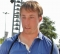Updated on 15-Jun-2020 15:46:19
Counting sort is a stable sorting technique, which is used to sort objects according to the keys that are small numbers. It counts the number of keys whose key values are same. This sorting technique is effective when the difference between different keys are not so big, otherwise, it can increase the space complexity.The complexity of counting Sort TechniqueTime Complexity: O(n+r)Space Complexity: O(n+r)Input and OutputInput: A list of unsorted data: 2 5 6 2 3 10 3 6 7 8 Output: Array before Sorting: 2 5 6 2 3 10 3 6 7 8 Array after Sorting: 2 2 3 ... Read More

## Comb Sort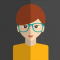Updated on 15-Jun-2020 14:29:38
The basic idea of comb sort and the bubble sort is same. In other words, comb sort is an improvement on the bubble sort. In the bubble sorting technique, the items are compared with the next item in each phase. But for the comb sort, the items are sorted in a specific gap. After completing each phase, the gap is decreased. The decreasing factor or the shrink factor for this sort is 1.3. It means that after completing each phase the gap is divided by 1.3.The complexity of Comb Sort TechniqueTime Complexity: O(n log n) for the best case. O(n^2/2^p) (p ... Read More

## Bucket Sort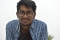Updated on 15-Jun-2020 14:35:08
In the Bucket Sorting technique, the data items are distributed in a set of buckets. Each bucket can hold a similar type of data. After distributing, each bucket is sorted using another sorting algorithm. After that, all elements are gathered on the main list to get the sorted form.The complexity of the Bucket Sort TechniqueTime Complexity: O(n + k) for best case and average case and O(n^2) for the worst case.Space Complexity: O(nk) for worst caseInput and OutputInput: A list of unsorted data: 0.25 0.36 0.58 0.41 0.29 0.22 0.45 0.79 0.01 0.69 Array before Sorting: 0.25 0.36 0.58 0.41 ... Read More

## Bubble SortUpdated on 15-Jun-2020 14:46:51
Bubble Sort is a comparison based sorting algorithm. In this algorithm adjacent elements are compared and swapped to make the correct sequence. This algorithm is simpler than other algorithms, but it has some drawbacks also. This algorithm is not suitable for a large number of data set. It takes much time to solve the sorting tasks.The complexity of the Bubble Sort TechniqueTime Complexity: O(n) for best case, O(n^2) for average and worst caseSpace Complexity: O(1)Input and OutputInput: A list of unsorted data: 56 98 78 12 30 51 Output: Array after Sorting: 12 30 51 56 78 98AlgorithmbubbleSort( array, size)Input ... Read More

## Ternary SearchUpdated on 15-Jun-2020 14:50:10
Like the binary search, it also separates the lists into sub-lists. This procedure divides the list into three parts using two intermediate mid values. As the lists are divided into more subdivisions, so it reduces the time to search a key value.The complexity of Ternary Search TechniqueTime Complexity: O(log3 n)Space Complexity: O(1)Input and OutputInput: A sorted list of data: 12 25 48 52 67 79 88 93 The search key 52 Output: Item found at location: 3AlgorithmternarySearch(array, start, end, key)Input − An sorted array, start and end location, and the search keyOutput − location of the key (if found), otherwise wrong ... Read More

## Linear Search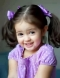Updated on 15-Jun-2020 14:54:10
Linear searching techniques are the simplest technique. In this technique, the items are searched one by one. This procedure is also applicable for unsorted data set. Linear search is also known as sequential search. It is named as linear because its time complexity is of the order of n O(n).The complexity of Linear Search TechniqueTime Complexity: O(n)Space Complexity: O(1)Input and OutputInput: A list of data: 20 4 89 75 10 23 45 69 the search key 10 Output: Item found at location: 4AlgorithmlinearSearch(array, size, key)Input − An sorted array, size of the array and the search keyOutput − location of the ... Read More

## Jump SearchUpdated on 15-Jun-2020 14:00:54
Jump search technique also works for ordered lists. It creates a block and tries to find the element in that block. If the item is not in the block, it shifts the entire block. The block size is based on the size of the list. If the size of the list is n then block size will be √n. After finding a correct block it finds the item using a linear search technique. The jump search lies between linear search and binary search according to its performance.The complexity of Jump Search TechniqueTime Complexity: O(√n)Space Complexity: O(1)Input and OutputInput: A sorted ... Read More

## Interpolation SearchUpdated on 15-Jun-2020 14:05:21
For the binary search technique, the lists are divided into equal parts. For the interpolation searching technique, the procedure will try to locate the exact position using interpolation formula. After finding the estimated location, it can separate the list using that location. As it tries to find exact location every time, so the searching time reduces. This technique can find items easily if the items are uniformly distributed.The complexity of Interpolation Search TechniqueTime Complexity: O(log2(log2 n)) for the average case, and O(n) for the worst case (when items are distributed exponentially)Space Complexity: O(1)Input and OutputInput: A sorted list of data: ... Read More

## Exponential Search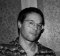Updated on 15-Jun-2020 14:10:42
Exponential search is also known as doubling or galloping search. This mechanism is used to find the range where the search key may present. If L and U are the upper and lower bound of the list, then L and U both are the power of 2. For the last section, the U is the last position of the list. For that reason, it is known as exponential.After finding the specific range, it uses the binary search technique to find the exact location of the search key.The complexity of Exponential Search TechniqueTime Complexity: O(1) for the best case. O(log2 i) ... Read More